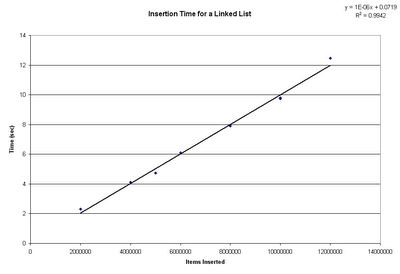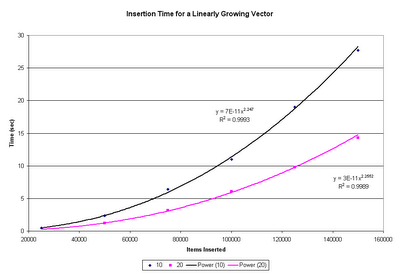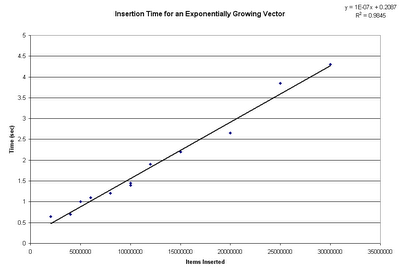## Saturday, June 03, 2006

### On Vectors and Lists

So, a brief follow-up post to the one about the flaw in GNUPlot: data structures 101. Well, not all data structures, just vectors and lists. I wrote a simple implementation of a vector and a linked list, then benchmarked them while adding a large (the definition of "large" varies by structure) number of items.

Your basic list is very simple: each item is allocated from the heap, and does not require copying of any existing data items at any time. Insertions take O(1), and a string of insertions take O(N), as illustrated by this benchmark:A vector is an array that gets resized whenever it gets full. When items are merely added to the end of an existing array, additions are O(1), and a series of additions are O(N). However, the process of resizing the array, which requires all existing items be copied, is O(N). This means that if you resize an array after a constant number of additions, additions to a vector will take O(N), and a string of additions will take O(N^2), as illustrated by this benchmark (the two series represent how much additional space was allocated each time the array was resized; 10 is the actual number GNUPlot uses when it reallocates its data array during file loading):This is the WRONG way to use a vector. The correct way is to increase the size of the vector exponentially. In my benchmark I used a factor of 2 - every time the array became filled, its size was doubled. Since it's growing exponentially, the number of allocations that must be done (each taking O(N)) will be O(1/2^N); O(N * 1/2^N) = 0. Thus the time to reallocate the array is negligible, and the complexity is determined strictly by the time to add each new elements to the already allocated array: O(1) per element, and O(N) for a series of elements. This is shown in the following graph: